﻿ Forecasting of Taiwan’s Gross Domestic Product using the Novel Nonlinear Grey Bernoulli Model with ANN Error Correction
Journal of Global Economics
• Research Article
• J Glob Econ 2016, Vol 4(1): 177
• DOI: 10.4172/2375-4389.1000177

# Forecasting of Taiwan’s Gross Domestic Product using the Novel Nonlinear Grey Bernoulli Model with ANN Error Correction

Chen CI1* and Hsin PH2
1Department of Industrial Management, I-Shou University, Taiwan
2Department of International Business, Cheng Shiu University, Taiwan
*Corresponding Author: Chen CI, Department of Industrial Management, I-Shou University, Taiwan, Tel: +886 7 6577711, Email: [email protected]

Received Date: Dec 11, 2015 / Accepted Date: Feb 09, 2016 / Published Date: Feb 12, 2016

### Abstract

Nonlinear Grey Bernoulli Model is proposed to enhance the prediction accuracy. In this study, artificial neural network (ANN) is used to modify the residual error of NGBM. Then, ANN error plus original forecasted value is a new estimated value. The newly proposed method termed NGBM (1,1) with ANN error correction is used to forecast Taiwan’s gross domestic product (GDP). The results show the proposed method is more accurate than NGBM and is proven to be effective in forecasting.

Keywords: Grey forecasting; Artificial neural network; Gross domestic product; Nonlinear Grey Bernoulli Model

#### Introduction

Grey theory  has been proposed over 30 years. Great endeavor has been devoted to increase the forecasting precision. One of methods treated the forecasting error to become the modified grey forecasting model. Hsu and Wen  modified original GM (1,1) models are improved by using residual modifications with Markov chain sign estimations. Hsu and Chen  improved grey GM (1,1) model, using a technique that combines residual modification with artificial neural network sign estimation, is proposed. Hsu  applied three residual modification models to enhance the forecasting results. The result showed the Markov-chain residual modification model achieves reliable and precise results. Zhou et al.  presented a trigonometric grey prediction approach by combining the traditional grey model GM (1,1) with the trigonometric residual modification technique for forecasting electricity demand. Jing  applied residual grey model (1,1) to the prediction of tuberculosis prevalence.

Above residual modification is based on linear GM (1,1) model. Recently, a nonlinear grey forecasting model which was termed NGBM was proposed. Chen et al.  proposed NGBM to forecast the foreign exchange rates of Taiwan’s major trading partners by novel nonlinear Grey Bernoulli model NGBM. Then, an improved NGBM model  was proposed to further increase the forecasting precision. Furthermore, Wang  used an optimized Nash nonlinear grey Bernoulli model for forecasting the main economic indices of high technology enterprises in China. Zhang  used a particle swarm optimization algorithm to solve the optimal parameter estimation problem, an improved Nash nonlinear grey Bernoulli model termed PSO-NNGBM (1,1) is proposed.

Through literature review, the NGBM with residual correction model has not been proposed. This study connects artificial neural network (ANN) with the Nonlinear Grey Bernoulli Model (NGBM) to develop a new hybrid Grey model, named NGBM with ANN error correction.

Gross domestic product (GDP) is the market value of all final goods and services produced within a country in a given period. It is often considered an indicator of a country’s standard of living. Besides, GDP is a sum of consumption, investment, government spending and net exports. Therefore, the consumers, the investors, the firms and the policy makers all care about the indicator of GDP. In this study, the proposed model is used to forecast Taiwan’s GDP. In testing period for 2011, the results indicate that the proposed model has a small estimated error. Besides, this study uses this method to forecast Taiwan’s GDP for 2012. The results show Taiwan is away from financial crisis and on the road to prosperity.

This paper is organized as follows. Section 2 introduces the mathematics of the Nonlinear Grey Bernoulli Model with ANN error correction, and defines the forecasting relative percentage error. Section 3 applies the proposed method to forecasting Taiwan’s GDP. Finally, Section 4 presents conclusions.

#### Methodology

The derivation of linear GM (1,1) and nonlinear NGBM are briefly introduced in the section. In order to increase the forecasting precision, the artificial neural network (ANN) is incorporated in the NGBM error correction model. The concept of ANN is also demonstrated.

The nonlinear grey Bernoulli model with ANN error correction

Step 1: Cpllect the original series of data with m entries is: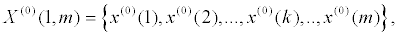(1)

where raw matrix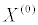represents the non-negative original time series data.

Step 2: Construct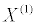using a one time accumulated generation operation (1-AGO), namely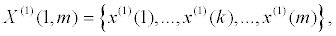(2)

Where,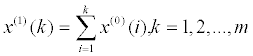(3)

Step 3: 1-AGO yields a monotonically increasing sequence similar to the solution curve of the first order linear differential equation. Therefore, the solution curve of the following differential equation approximates the 1-AGO data.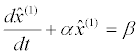(4)

Where, ^ represents the Grey predicted value. The α and β are model parameters;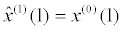is the initial condition.

Step 4: Model parameters α and β can be calculated by discretization of Eq. (4)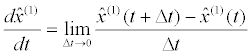(5)

let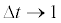, in which case 1-AGO approximates the forecast value,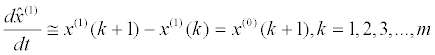(6)the background value, is defined as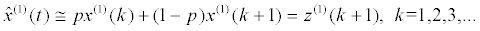(7)

Where, the production coefficient p is in the range 0-1, and traditionally equals 0.5. Thus, the source model can be obtained as follows: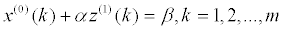(8)

The model parameters α and β can be solved by least square methods.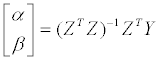(9)

where Z and Y are defined as follows: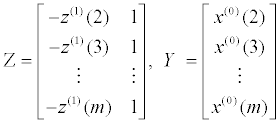(10)

Step 5: Solve Eq. (4) together with the initial condition, and the particular solution is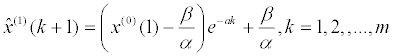(11)

Consequently, the prediction output at step k can be estimated using the inverse accumulated generating operation (IAGO). It is defined as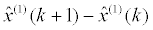(12)

or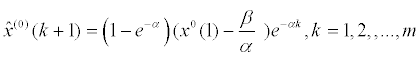(13)

Step 6: Equation (4) is linear differential equation and the only adjustable variable is background value p. Based on the elementary course in ordinary differential equation, a similar form of differential equation to eq. (4) is called Bernoulli equation , which is nonlinear and has the following form,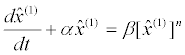(14)

Where, n belongs to any real number except one. There are two special cases of NGBM. When n=0, the equation is original grey forecasting model. When n=2, the equation is Grey-Verhulst equation .

Step 7: A discrete form of eq. (14) is described as.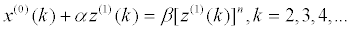(15)

By least square method, the above model parameters a and b become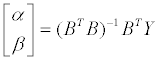(16)

where B and Y are defined as follows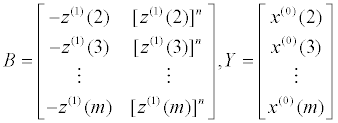(17)

Step 8: The corresponding particular solution of eq. (14) is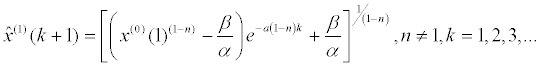(18)

Step 9: Take the inverse accumulated generation operation (IAGO) on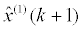to obtain the estimated value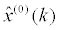IAGO is defined in eq (12).

The error between forecasting and raw data is inevitable; therefore, the minimization of error is one approach to increase forecasting precision. By the conception of error correction, this study proposes a novel grey model, named NGBM with ANN error correction. The original forecast value is obtained by NGBM; the estimated forecast error is calculated by ANN. Finally, the original forecast value plus the estimated forecast error is the proposed forecast value. The procedure for obtaining ANN error correction is described as follows.

Step 10: One of modified residual GM (1,1) model was proposed by Deng . In this research, the ANN residual model is proposed. The differences between the real

values,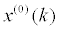,and the forecasted values,are defined as the residual series. The residual series is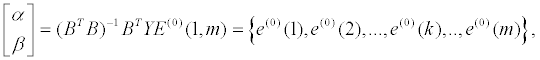(19)

where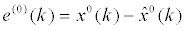Step 11: Let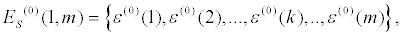Where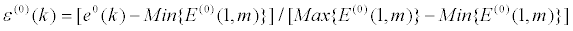(20)

Note that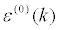is a standardized error.

Step 12: Use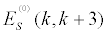to forecast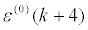by ANN. The parameters about ANN are described in section 3.

Step 13: Thus, the forecasted values is defined as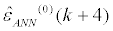Step 14: Calculate the forecasting value with ANN error correction. Here, it is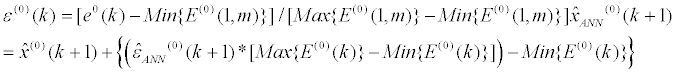(21)

Measures of forecasting performance

The residual error test is the main criterion for assessing prediction accuracy. Grey forecasting usually uses (average) relative percentage error to examine the forecast precision. Generally speaking, the model which has small residual error is regard as a good model. The relative percentage error (RPE) and theaverage relative percentage error (ARPE) are defined as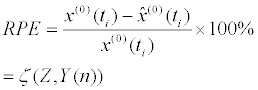(22)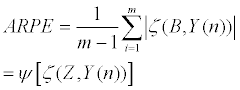(23)

#### Forecasting Taiwan's GDP

In this study, Taiwan’s GDP is used to verify the proposed model. Data is from Ministry of Economic Affairs  in Taiwan. GDP is the market value of all of the final goods and services produced within the country’s borders in a one-year period. GDP can be determined in three ways which are the product approach, the income approach, and the expenditure approach. In principle, they have the same result. The expenditure approach method is broadly used and easy to calculate. GDP (Y) is a sum of consumption (C), investment (I), government spending (G) and net exports (X – M). That is, Y = C + I + G + (X − M). C (consumption) is usually the largest GDP component in the economy, consisting of household final consumption expenditure in the economy. I (investment) contain business investment in fixtures and equipment, but do not include exchanges of existing assets. G (government spending) is the sum of government expenditures on final goods and services. It comprises salaries of public servants, purchase of weapons for the military, and any investment expenditure by a government. X (exports) expresses gross exports. GDP measures the amount a country produces, including goods and services produced for other nations’ consumption, therefore exports are added. M (imports) expresses gross imports. Imports are subtracted since imported goods will be included in the terms G, I, or C, and must be deducted to avoid counting foreign supply as domestic.

Data from 2004 to 2010 is used to construct the proposed model. And then forecast Taiwan’s GDP for 2011 to verify the proposed model. Every series containing four data is regard as inputs and the firth data is output.

Empirical results of NGBM (1,1)

The process of obtaining the forecasted values by NGBM (1,1) is described in the following.

(1) Denote the original data series,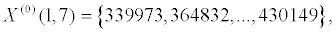(2) Thus, the AGO series of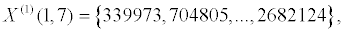(3) And, the background value series are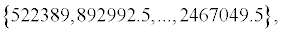(4) After constructing the matrices Z and Y, solve the following objective function.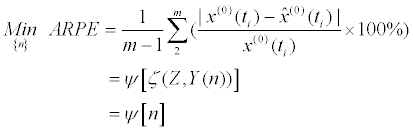By Newtonian method, n is 0.03 and ARPE is 2.3289

(5) The estimated value series by NGBM are listed in Table 1.

(6) For forecasting GDP of 2012, the data of 2011 is treated as new information. So, the new original data series is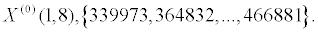After repeating above procedure, the estimated value of 2012,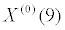is 430647.04 (n = -0.06 and ARPE=2.5484). The above results are showed in Table 2.

Year Actual value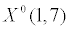NGBM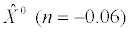Proposed model
Forecasted values RPE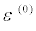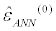Forecasted values RPE*
2004 339973 339973.00 0.0000 0.6217
2005 364832 364364.88 0.0013 0.6323
2006 376375 376349.30 0.0001 0.6222
2007 393134 386512.99 0.0168 0.7725
2008 400132 395877.79 0.0106 0.7186 0.6114 395427.02 0.0118
2009 377529 404824.19 -0.0723 0.0000 0.1934 386020.51 -0.0225
2010 430149 413537.71 0.0386 1.0000 0.9269 426939.44 0.0075
2011 466881 422123.43 0.0959   0.9947 438502.02 0.0608

Note: *RPE is relative percentage error as defined in eq(22);is the standardized residual error,ANN ε the estimated standardized residual error by ANN.

Table 1: Actual value, forecasted value, and relative percentage error for Taiwan’s GDP by NGBM(1,1) and the proposed model (Units: US\$ million)

Year Actual value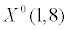NGBMProposed model
Forecasted values RPEForecasted values RPE*
2004 339973 339973.00 0.0000 0.3788
2005 364832 364529.18 0.0830 0.3853
2006 376375 371690.68 1.2446 0.3792
2007 393134 383328.73 2.4941 0.4707
2008 400132 397400.64 0.6826 0.4379 0.3268 392129.44 0.0200
2009 377529 413236.91 -9.4583 0.0000 0.2172 393178.85 -0.0415
2010 430149 430554.33 -0.0942 0.6094 0.5694 427269.35 0.0067
2011 466881 449222.68 3.7822 1.0000 0.9254 461505.86 0.0115
2012   469185.25     0.8075 461534.45 /

Note: *RPE is relative percentage error as defined in eq (22);is the standardized residual error,ANN ε the estimated standardized residual error by ANN.

Table 2: Actual value, forecasted value, and relative percentage error for Taiwan’s GDP by NGBM(1,1) and the proposed model (Units: US\$ million)

Empirical results of NGBM (1,1) with ANN error correction

Since forecast error can’t be avoided, the researcher can try to estimate the forecast error in advance and then correct them on original estimated value. The process of obtaining the forecasting value by NGBM (1,1) with ANN Error Correction is described in the following.

(1) Denote the original forecasting error series,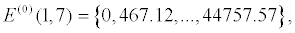(2) Calculate the standardized errorby eq.(20).

(3) The modeling period is from 2004 to 2010. Useto forecastby BP ANN. Therefore, there are three error series used for BP ANN. The parameters for computer program of Mat lab are

LP.lr = 0.1;

net.trainParam.epochs = 15000;

net.trainParam.goal = 0.025;

(4) The testing year is 2011 and the estimated GDP is 438502.02. The above results are showed in Table 2.

(5) For forecasting GDP of 2012, the new forecasting error series is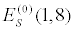S E . After repeating above procedure, the estimated value of GDP is 461534.45. The above results are showed in Table 2.

Comparisons of forecasting performance

The error measures used for the forecasting comparison are the ARPE for in-sample data (2008-2010) and for out-of sample data (2011). Form Table 1, the RPE of NGBM (1,1) from 2008 to 2010 are 0.0106, -0.0723 and 0.0386; the RPE of NGBM (1,1) with error correction from 2008 to 2010 are, 0.0118, 0.0225 and 0.0075. Thus the former ARPE is. 0.0405; the latter ARPE is 0.0139. The model fitting results indicates NGBM (1,1) with error correction has lower forecast error.

Comparing the out-of sample data (2011), the RPE of NGBM (1,1) is 0.0959; the RPE of NGBM(1,1) with error correction is 0.0608. According to the results, the newly proposed model, NGBM (1,1) with error correction, is with a highly forecasting accuracy.

To forecast the GDP of 2012, the data of 2011 have to inject into the grey forecasting system. As a results, the modeling error for NGBM (2008-2011) are 0.6826, -9.4583, -0.0942 and 3.7822; the RPE of the proposed model (2008-2011) are, 0.0200, -0.0415, 0.0067 and 0.0115. Thus the former ARPE is. 3.5043; the latter ARPE is 0.0199. The model fitting results also indicates the proposed model has lower forecast error.

The more accurate forecasting of future GDP trends is clearly of significant importance to both government and company financial directors. Both NGBM (1,1)and the proposed model show that Taiwan is on the road to prosperity and away from financial meltdown.

#### Conclusions

Grey forecasting only requires a small data and make perfect in dealing with incomplete and uncertain information. Thus, grey forecasting is suitable for forecasting GDP. Among grey forecasting, NGBM (1,1) have been proven with high forecasting accuracy. This study proposes a hybrid grey forecasting, named NGBM (1,1) with ANN error correction for Taiwan’s GDP forecast. The idea of correcting forecast error in advance makes the proposed method useful in enhancing forecasting accuracy. In sample testing and out-of sample testing, the results support above contention.

#### References

Citation: Chen CI, Hsin PH (2016) Forecasting of Taiwan’s Gross Domestic Product using the Novel Nonlinear Grey Bernoulli Model with ANN Error Correction. J Glob Econ 4: 177. Doi: 10.4172/2375-4389.1000177

Copyright: © 2016 Chen CI, et al. This is an open-access article distributed under the terms of the Creative Commons Attribution License, which permits unrestricted use, distribution, and reproduction in any medium, provided the original author and source are credited.

Select your language of interest to view the total content in your interested language

Rome, Italy

Vienna, Austria

### 5th World Dermatology and Aesthetic Congress

Capetown, South Africa
##### Recommended Journals
Viewmore
###### Article Usage
• Total views: 9227
• [From(publication date): 3-2016 - Jan 24, 2020]
• Breakdown by view type
• HTML page views: 9085Can't read the image? click here to refresh